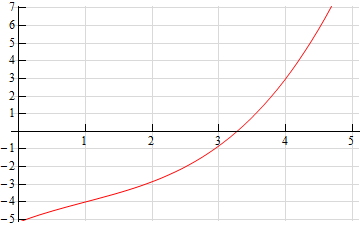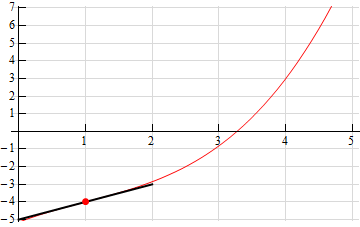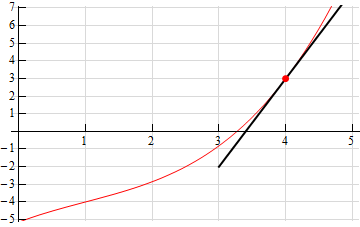Paul's Online Notes
Home / Calculus I / Derivatives / Interpretation of the Derivative
Show Mobile Notice Show All Notes Hide All Notes
Mobile Notice
You appear to be on a device with a "narrow" screen width (i.e. you are probably on a mobile phone). Due to the nature of the mathematics on this site it is best views in landscape mode. If your device is not in landscape mode many of the equations will run off the side of your device (should be able to scroll to see them) and some of the menu items will be cut off due to the narrow screen width.

### Section 3.2 : Interpretation of the Derivative

2. Use the graph of the function, $$f\left( x \right)$$, estimate the value of $$f'\left( a \right)$$ for

1. $$a = 1$$
2. $$a = 4$$Hint : Remember that one of the interpretations of the derivative is the slope of the tangent line to the function.

Show All Solutions Hide All Solutions

a $$a = 1$$ Show All Steps Hide All Steps
Start Solution

Given that one of the interpretations of the derivative is that it is the slope of the tangent line to the function at a particular point let’s first sketch in a tangent line at the point on the graph.Show Step 2

The function is clearly increasing here and so we know that the derivative at this point will be positive. Now, from this sketch of the tangent line it looks like if we run over 1 we go up 1 and so we can estimate that,

$\require{bbox} \bbox[2pt,border:1px solid black]{{f'\left( 1 \right) = 1}}$

b $$a = 4$$ Show All Steps Hide All Steps
Start Solution

Given that one of the interpretations of the derivative is that it is the slope of the tangent line to the function at a particular point. Let’s first sketch in a tangent line at the point.Show Step 2

The function is clearly increasing here and so we know that the derivative at this point will be positive. Now, from this sketch of the tangent line it looks like if we run over 1 we go up 5 and so we can estimate that,

$\require{bbox} \bbox[2pt,border:1px solid black]{{f'\left( 4 \right) = 5}}$